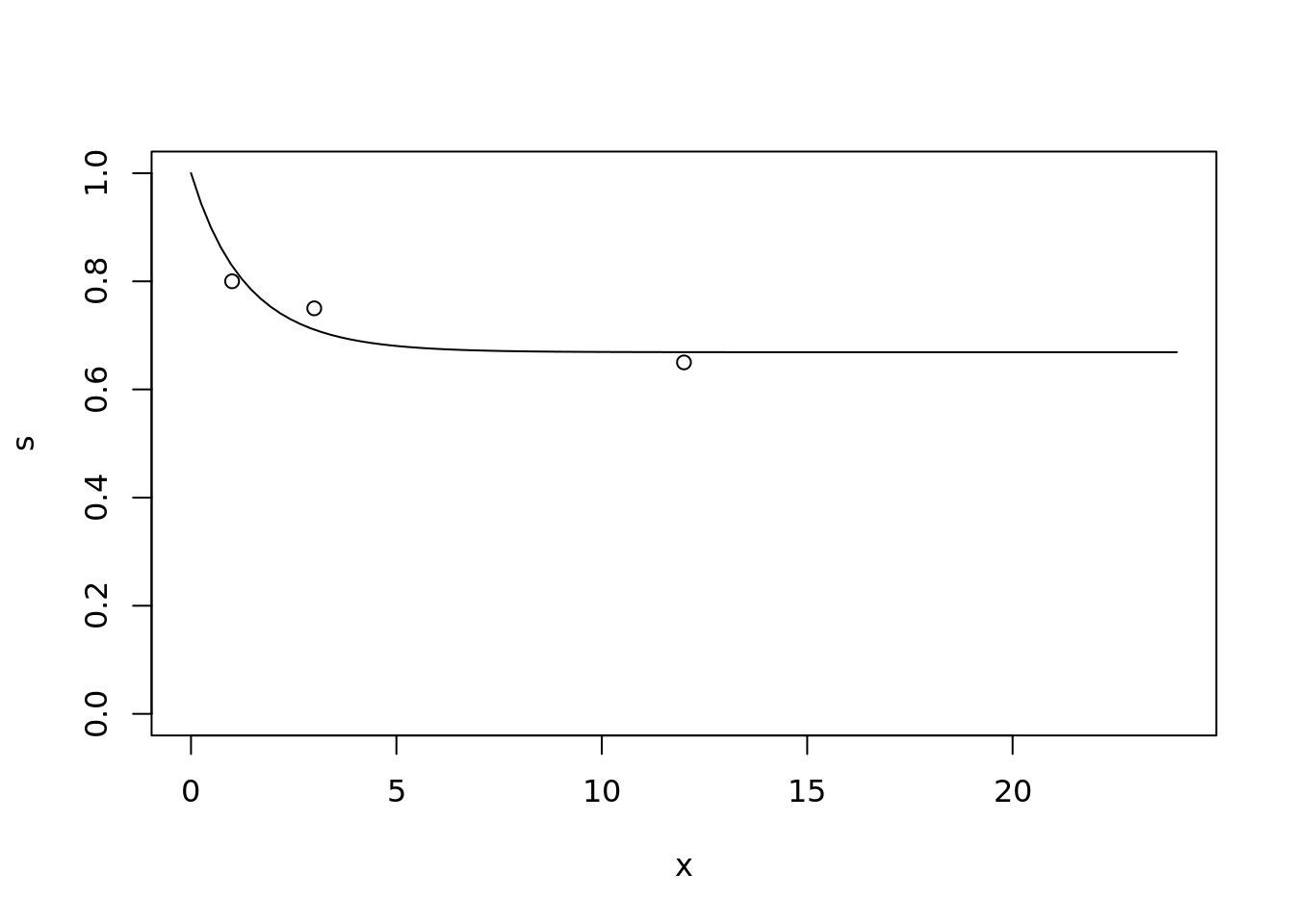# That'll do: simulated annealing instead of maths

This is a post for future me. Given my steadily declining maths skills, I can see myself needing to use the generalized simulated annealing function GenSA::GenSA a lot more.

For example, suppose I’m helping to design a clinical trial and I want to simulate some data from a Gompertz distribution. I know that the Gompertz distribution allows a rapidly decreasing hazard function (that’s why I want to use it), but I’m not familiar with the details, and don’t have the time/motivation right now to do any maths. I want the survival probability at 1 month to be about 80%, at 3 months to be about 75%, and at 12 months to be about 65%. What are the corresponding parameters of the Gompertz distribution?

From looking at the help pages ?flexsurv::pgompertz, i see that the Gompertz has a shape parameter a and a rate parameter b. I write a quick function to calculate the distance between a Gompertz(a,b) distribution and my desired milestone probabilities:

gomp_close <- function(a_b,
t_1, ## timepoint 1
t_2, ## timepoint 1
t_3, ## timepoint 2
p_1, ## p(event by t_1)
p_2,
p_3){

a <- a_b
b <- a_b

distance_1 <- flexsurv::pgompertz(t_1, a, b) - p_1
distance_2 <- flexsurv::pgompertz(t_2, a, b) - p_2
distance_3 <- flexsurv::pgompertz(t_3, a, b) - p_3

distance_1 ^ 2 + distance_2 ^ 2 + distance_3 ^ 2

}

and then I let the simulated annealing function find a close enough solution:

result <- GenSA::GenSA(par = c(1, 1), # initial values
fn = gomp_close,
lower = c(-100, 0), # parameter b is positive, so lower lim 0
upper = c(100, 100),
t_1 = 1,
t_2 = 3,
t_3 = 12,
p_1 = 0.2,
p_2 = 0.25,
p_3 = 0.35)

result$par ##  -0.6307335 0.2536741 Just check this looks ok: x <- seq(0, 24, length.out = 100) s <- flexsurv::pgompertz(x, shape = result$par,
rate = result\$par,
lower.tail = FALSE)

plot(x, s, type = "l", ylim = c(0,1))
points(c(1, 3, 12), c(0.8, 0.75, 0.65))##### Dominic Magirr
###### Medical Statistician

Interested in the design, analysis and interpretation of clinical trials.